## Monday, March 13, 2017

This should be a long post, but I am going to try and keep it as brief as possible. This post is more like an excerpt from the publication 'Structural Analysis and Design of Residential Buildings using Staad Pro V8i, CSC Orion, and Manual calculations'.... (See link below).

Here, we are going to briefly present some practical analysis and design of some reinforced concrete elements using Staad Pro software, Orion software and manual calculations.
Ultimately, we are going to make some comparisons of the results obtained based on the different methods adopted in the analysis and design. To learn how to model, design, and detail buildings from the scratch using Staad Pro, Orion, and manual methods, see the link at the end of this post.

To show how this is done, a simplified architectural floor plans, elevations, and section, for a residential two storey building have been presented for the purpose of structural analysis and design (see the pictures below).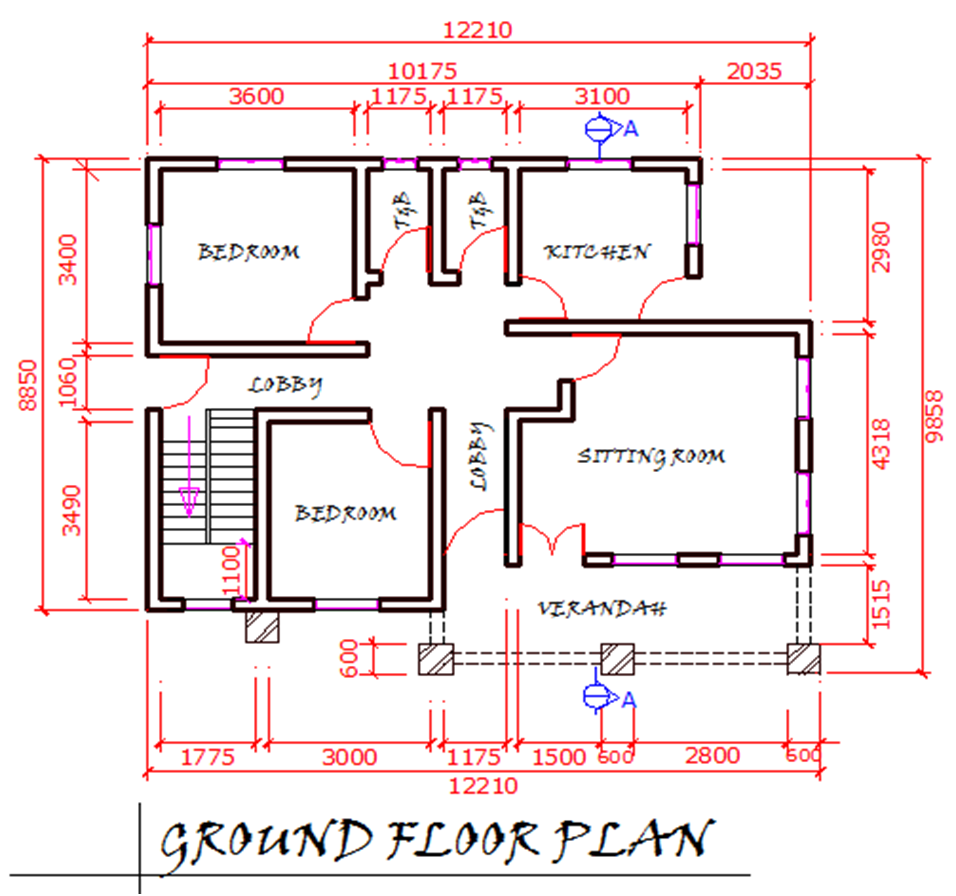Fig 1: Ground Floor PlanFig.2: First Floor PlanFig.3: Front ViewFig.4: Back ViewFig.5: Right ViewFig.6: Left View

The first step in the design of buildings is the preparation of the 'general arrangement', popularly called the G.A. The G.A. is a drawing that shows the disposition of the structural elements such as the slabs and their types, the floor beams, the columns, and their interaction at the floor level under consideration. For the architectural drawings above, the adopted G.A. is shown in Figure 7. below. There are no spelt out rules about how to prepare G.A. from architectural drawings, but there are basic guidelines that can guide someone on how to prepare a buildable and structurally efficient G.A. To have a good idea on how this can be done, see the link at the end of the post.

Fig.7: General Arrangement

Design data:
Fck = 25 N/mm2, Fyk = 460 N/mm2, Cnom (slabs) = 25mm, Cnom (beams and columns) = 35mm, Cnom (foundations) = 50mm
Thickness of slab = 150mm; Dimension of floor beams = 450mm x 230mm; Dimension of columns = (230 x 230mm)

DESIGN OF THE FLOOR SLABS
PANEL 1: MANUAL ANALYSIS
The floor slab (PANEL 1) is spanning in two directions, since the ratio (k) of the longer side (Ly) to the shorter side (Lx) is less than 2.
Hence, k = Ly/Lx = 3.825/3.625 = 1.055 (say 1.1)

Moment coefficients (α) for two adjacent edges discontinuous (pick from table);
Short Span
Mid-span = 0.042
Continuous edge = 0.056
Long Span
Mid-span = 0.034
Continuous edge = 0.045

Design of short span
Mid span
M = αnLx2 = 0.042 × 10.9575 × 3.6252 = 6.0475 KN.m
MEd = 6.0475 KNm
Effective Depth (d) = h – Cc – ϕ/2
Assuming ϕ12mm bars will be employed for the construction
d = 150 – 25 – 6 = 119mm; b = 1000mm (designing per unit width)

k = MEd/(fckbd2 )
= (6.0475 × 106)/(25 × 1000 × 1192 ) = 0.0171
Since k < 0.167 No compression reinforcement required
z = d[0.5+ √(0.25 - 0.882k)] = z = d[0.5+ √(0.25 - (0.882 × 0.0273)] = 0.95d
As1 = MEd/(0.87fyk z)
As1 = (6.0475 × 106)/(0.87 × 460 × 0.95 × 119) = 133.668 mm2/m
Provide Y12mm @ 250mm c/c BOT (ASprov = 452 mm2/m)

A little consideration will show that this provided area of steel will satisfy serviceability limit state requirements. To see how to carry out deflections and crack control verifications, see the the link at the bottom of this post.

Result from Orion showing the Short Span (mid span) design moments (Wood and Armer effects inclusive) (PANEL 1)

Result from Staad showing the Short Span (mid span) design moments (Wood and Armer effects inclusive) (PANEL 1)

A little observation will show that the design moment values from the different methods are very similar. The full detailing of the floor slabs is as shown below.

Figure 9: Bottom Reinforcement Detailing

Figure 10: Top Reinforcement Detailing

Figure 11: Section of the floor slab

DESIGN OF THE BEAMS
Let us take Beam No 1 from our GA as a design case study:
The loading of the beam has been carried out as shown below. The beam is primarily subjected to load from slab, weight of wall, and its own self weight. To see how to manually calculate the loading on beams, follow the link at the end of the post.

The internal forces from Orion software for Beam No 1 is as shown below. Load decomposition using finite element analysis was used for the load transfer.

The internal forces from Staad software for Beam No 1 is as shown below.

As1 = MEd/(0.87fykz)
= (36.66 × 106)/(0.87 × 460 × 0.95 × 399) = 241.667 mm2
Provide 2Y16 mm BOT (ASprov = 402 mm2)
The detailing of Beam No 1 is as shown below;

DESIGN OF THE COLUMNS
Loads from slabs and beams are transferred to the foundations through the columns. In typical cases, columns are usually rectangular or circular in shape. Normally, they are usually classified as short or slender depending on their slenderness ratio, and this in turn influences their mode of failure. Columns are either subjected to axial, uniaxial, or biaxial loads depending on the location and/or loading condition. Eurocode 2 demands that we include the effects of imperfections in structural design of columns. Column design is covered in section 5.8 of EC2.

The column axial loads have been obtained by summing up the reactions from all the beams supported by the columns, including the self weight of the column. Let us use column A1 as example. At the roof level, the column is supporting beam No 2 (Support Reaction V1 = 13.27 KN) and Beam No 3 (Support Reaction VA = 12.99 KN). At the first floor level (see Analysis and Design of Beam No 1 and 2), the column is supporting Beam No 1 (Support Reaction V1 = 41.38 KN), and Beam No 2 (Support Reaction VA = 42.49 KN). Therefore the summation of all these loads gives the axial load transferred from the beams. For intermediate supports, note that the summation of the shear forces at the support gives the total support reaction (neglect the signs and use absolute value. Another method of calculating Column Axial Load is by Tributary Area Method. This method has not been adopted in this work.

COLUMN A1
Total Columns Self weight = 12.14 KN
Load from roof beams = 13.27 + 12.99 = 26.26 KN
Load from floor beams = 46.21 + 42.49 = 88.70 KN
Total = 127.13 KN

Axial Load from Orion (A1) = 126.6 KN

COLUMN A3
Total Columns Self weight = 12.14 KN
Load from roof beams = 35.41 + 11.46 = 46.87 KN
Load from floor beams = 105.33 + 60.85 = 166.18 KN
Total = 225.19 KN

Axial Load from Orion (A3) = 202.3 KN

COLUMN A5
Total Columns Self weight = 12.14 KN
Load from roof beams = 17.19 + 5.70 = 22.89 KN
Load from floor beams = 83.64 + 37.91 = 121.55 KN
Total = 156.58 KN

Axial Load from Orion (A5) = 155.9 KN

COLUMN A7
Total Columns Self weight = 12.14 KN
Load from roof beams = 43.15 + 9.48 = 52.63 KN
Load from floor beams = 38.26 + 62.45 = 100.71 KN
Total = 165.48 KN

Axial Load from Orion (A7) = 133.9 KN

As you can see, for design purposes, the axial loads from the three methods are very comparable. To see how to obtain the column design moments from the use of sub-frames, follow the link at the end of the post.

Design of Column E5
Reading from chart; d2/h = 0.2;
MEd/(fck bh2 )
= (10.002 × 106)/(25 × 230 × 2302 ) = 0.03288
NEd/(fckbh)
= (399.88 × 103)/(25 ×230 × 230) = 0.302
From the chart:
(AsFyk)/(bhfck ) = 0.05
Area of longitudinal steel required (As) = (0.05 × 25 × 230 × 230)/460 = 143.75 mm2
As,min = 0.10 NEd/fyd
= (0.1 × 399.887)/400 = 0.099 mm2 < 0.002 × 230 × 230 = 105.8 mm2
Provide 4Y16mm (Asprov = 804 mm2)

Minimum size = 0.25ϕ = 0.25 × 16 = 4mm < 6mm
Spacing adopted = 200mm less than min{b, h, 20ϕ, 400mm}

Result from Orion for column E5

Result from Staad for column E5

The column detailing is as shown below;

DESIGN OF FOUNDATIONS
All loads from the superstructure of a building are transferred to the ground. If the foundation of a building is poorly designed, then all the efforts input in designing the superstructure is in vain. It is therefore imperative that adequate care be taken in the design of foundations. Foundation design starts from detailed field and soil investigation. It is very important to know the index and geotechnical properties of the soil, including the soil chemistry, so that the performance of the foundation can be guaranteed.

Analysis and Design of footing E8

Bearing Capacity of the foundation = 150 KN/m2;

Effective depth
Concrete cover = 50mm
AssumingY12mm bars,
d = 400 – 50 – 6 = 344mm
The ultimate limit state design moment can be obtained by considering the figure below;

k = MEd/(fck bd2)
= (37.518 × 106)/(25 × 1000 × 3442 ) = 0.01268 (designing per metre strip)
Since k < 0.167 No compression reinforcement required
z = d[0.5+ √(0.25 - 0.882k)] = z = d[0.5+ √(0.25 - (0.882 × 0.0273)] = 0.95d
As1 = MEd/(0.87fykz)
= (37.518 × 106)/(0.87 × 460 × 0.95 × 344) = 286.869 mm2/m

To calculate the minimum area of steel required;
fctm = 0.3 × (fck)(2⁄3) = 0.3 × 25 (2⁄3) = 2.5649 N/mm2 (Table 3.1 EC2)
ASmin = 0.26 × fctm/Fyk × b × d = 0.26 × 2.5649/460 ×1000 × 344 = 498.7 mm2
Check if ASmin < 0.0013 × b × d (447.2 mm2)
Since, ASmin = 498.7 mm2, the provided reinforcement is adequate.
Provide Y12 @ 200mm c/c (ASprov = 565 mm2/m) each way

Shear at the column face
Ultimate Load on footing from column = 399.887 kN
Design shear stress at the column perimeter vEd = βVEd/(u0d)
β is the eccentricity factor (see section 6.4.3 of EC2)
β = 1+ 1.8√[(16.48/230)2+(8.99/230)2] = 1.146
Where uo is the column perimeter and d is the effective depth
vEd = βVEd/(u0d)
= (1.15 × 399.887 × 103)/(4(230) × 344) = 1.452N/mm2
VRd,max = 0.5vfcd
v = 0.6[1 – (fck/250) ] = 0.6[1 – (25/250) ] = 0.54 N/mm2
fcd = (αcc fck)/γc = (0.85 × 25)/1.5 = 14.167 N/mm2
VRd,max = 0.5 × 0.54 × 14.167 = 3.825 N/mm2 vEd < VRd,max. This is very ok

Transverse shear at ‘d’ from the face of column
Width of shaded area = a – d = 0.635 – 0.344 = 0.291m
Area of shaded area = (1.5m × 0.291m) = 0.4365 m2
Therefore, ΔVEd = (189.386 + 175.939)/2 × 0.4365 m2 = 79.077 KN
vEd = VEd/bd
= (79.077 × 103)/(1500 × 344) = 0.15325 N/mm2
VRd,c = [CRd,c k (100ρ1 fck )(1/3) + k1cp] × (2d/a) ≥ (Vmin + k1.σ,sub>cp) bw.d
CRd,c = 0.18/γc = 0.18/1.5 = 0.12
k = 1+√(200/d) = 1+√(200/344) = 1.7624 > 2.0, therefore, k = 1.7624
Vmin = 0.035k(3/2) fck(1/2)
= Vmin = 0.035 × (1.7624)(3/2) × (25)(1/2) = 0.4094 N/mm2
ρ1 = As/bd = 565/(1000 × 344) = 0.001642 < 0.02;
VRd,c = [0.12 × 1.7624 (100 × 0.001642 ×25 )(1/3) ] = 0.3386 N/mm2 × (2d/a) < Vmin
But in this case, d = a
Hence, VRd,c = 2 × 0.3386 = 0.6772 N/mm2
Since VRd,c (0.6772) > VEd (0.1503 KN), No shear reinforcement is required.

Punching Shear at 2d from the face of column
Punching shear lies outside the footing dimensions. No further check required.

Design Result from Orion

The detailing of the footing is as shown below;

The structural analysis and design of all members have been fully done, including a step by step tutorial on how to model and design on Orion and Staad Pro, and how to manually design. See the completed models below;

Fully completed model on Orion

Fully completed model on Staad Pro.

To download the simplified e-book where all the members have been designed and completely detailed, including bar bending schedule and quantification of materials, click HERE.

Thank you, and God bless you.

1.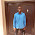Well done sir

2.What is c nom

1.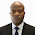Nominal cover to reinforcement (concrete cover)

3.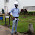In short God Bless you my able Engr., I really love your effort and I also pray for myself to have a such zeal to help other like this.

1.Thank you very much... We will keep working hard

2.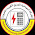thanks engineer Ubani and this is a big effort i hope to you full successful in your life
Regards..

4.your have great zeal ....i wish you goodlucky in future....how can i get your practical book.....

5.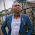my broda God bless u. u r doing a great work, d lord is ur strength.

1.Amen... Thank you bro

6.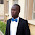You have done well..... How can I purchase that book...

7.Well-done sir, but I can't find any link in the end

8.Sir I finished in a school were by we cram to pass now am a graduate what will I show for outside I really need to be good at something

1.You now have to discover yourself based on what you really want, and give your all towards getting it.

9.good job, but one thing still unclear, how did you arrive at 12.147KN has column self weight?? by my calculation its 0.23*0.23*3*24=3.8KN

10.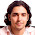If you want your residential construction business to be a success, it is vital that you have solid record keeping procedures and immaculate books: If the numbers aren't right the whole business could go under. room addition los angeles

11.12.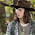13.I love significantly your own post! I look at all post is great. I discovered your personal content using bing search. Discover my webpage is a great one as you.I work to create several content this post. Once more you can thank you and keep it create! Enjoy! "green" paints & stains

14.15.God bless you Sir. U did it all .

16.Thanks for this straight forward analysis,but sir how can someone load a Column of more than 2 floors

17.Great Job.
Thank You Sir